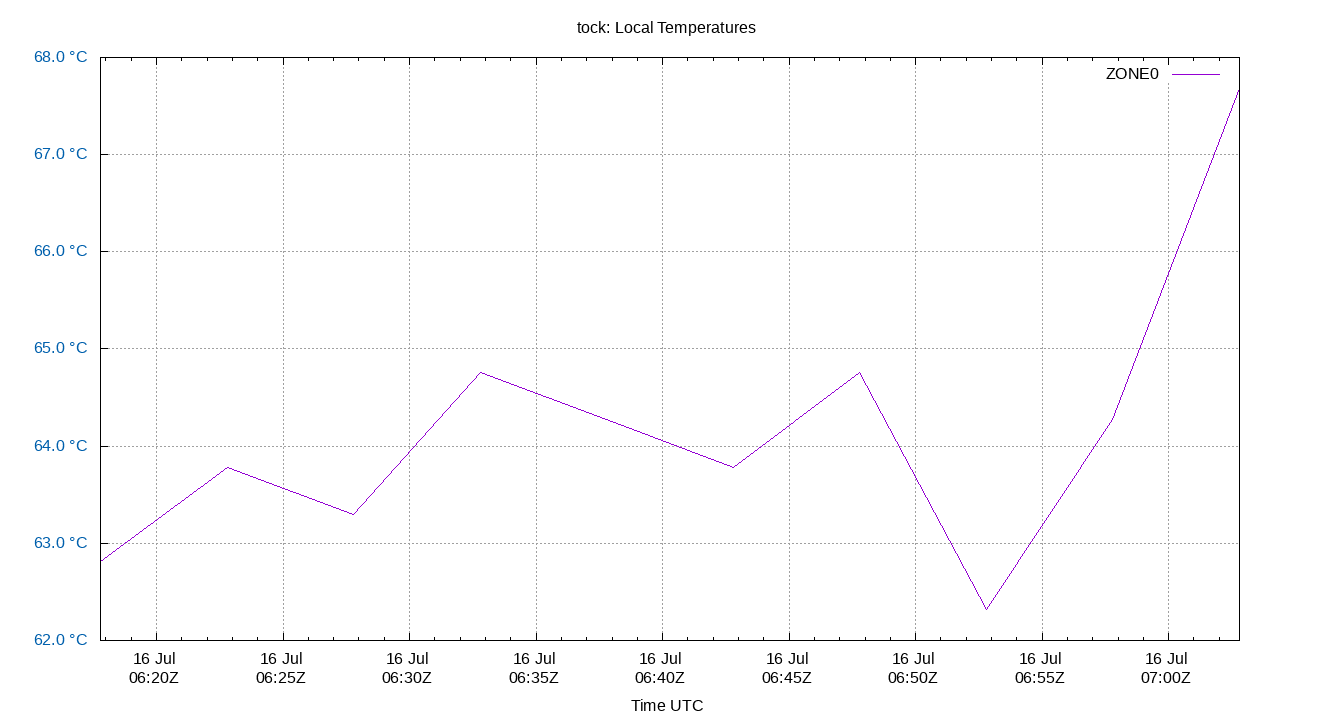# tock

Report generated: Wed Mar 22 13:15:18 2023 UTC
Start Time: Tue Mar 21 13:15:18 2023 UTC
End Time: Wed Mar 22 13:15:18 2023 UTC
Report Period: 1.0 days

## Local TemperaturesLocal temperatures. These will be site-specific depending upon what temperature sensors you collect data from. Temperature changes affect the local clock crystal frequency and stability. The math of how temperature changes frequency is complex, and also depends on crystal aging. So there is no easy way to correct for it in software. This is the single most important component of frequency drift.

The Local Temperatures are from field 3 from the tempstats log file.

## Summary

 Percentiles...... Ranges...... Skew- Kurt- Name Min 1% 5% 50% 95% 99% Max 90% 98% StdDev Mean Units ness osis
Summary as CSV file

## Glossary:

frequency offset:
The difference between the ntpd calculated frequency and the local system clock frequency (usually in parts per million, ppm)
jitter, dispersion:
The short term change in a value. NTP measures Local Time Jitter, Refclock Jitter, and Server Jitter in seconds. Local Frequency Jitter is in ppm or ppb.
kurtosis, Kurt:
The kurtosis of a random variable X is the fourth standardized moment and is a dimension-less ratio. ntpviz uses the Pearson's moment coefficient of kurtosis. A normal distribution has a kurtosis of three. NIST describes a kurtosis over three as "heavy tailed" and one under three as "light tailed".
ms, millisecond:
One thousandth of a second = 0.001 seconds, 1e-3 seconds
mu, mean:
The arithmetic mean: the sum of all the values divided by the number of values. The formula for mu is: "mu = (∑xi) / N". Where xi denotes the data points and N is the number of data points.
ns, nanosecond:
One billionth of a second, also one thousandth of a microsecond, 0.000000001 seconds and 1e-9 seconds.
percentile:
The value below which a given percentage of values fall.
ppb, parts per billion:
Ratio between two values. These following are all the same: 1 ppb, one in one billion, 1/1,000,000,000, 0.000,000,001, 1e-9 and 0.000,000,1%
ppm, parts per million:
Ratio between two values. These following are all the same: 1 ppm, one in one million, 1/1,000,000, 0.000,001, and 0.000,1%
‰, parts per thousand:
Ratio between two values. These following are all the same: 1 ‰. one in one thousand, 1/1,000, 0.001, and 0.1%
refclock:
Reference clock, a local GPS module or other local source of time.
remote clock:
Any clock reached over the network, LAN or WAN. Also called a peer or server.
time offset:
The difference between the ntpd calculated time and the local system clock's time. Also called phase offset.
σ, sigma:
Sigma denotes the standard deviation (SD) and is centered on the arithmetic mean of the data set. The SD is simply the square root of the variance of the data set. Two sigma is simply twice the standard deviation. Three sigma is three times sigma. Smaller is better.
The formula for sigma is: "σ = √[ ∑(xi-mu)^2 / N ]". Where xi denotes the data points and N is the number of data points.
skewness, Skew:
The skewness of a random variable X is the third standardized moment and is a dimension-less ratio. ntpviz uses the Pearson's moment coefficient of skewness. Wikipedia describes it best: "The qualitative interpretation of the skew is complicated and unintuitive."
A normal distribution has a skewness of zero.
upstream clock:
Any server or reference clock used as a source of time.
µs, us, microsecond:
One millionth of a second, also one thousandth of a millisecond, 0.000,001 seconds, and 1e-6 seconds.